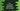# C++ iswupper() method explanation with example## C++ iswupper() method explanation with example:

iswupper is used to check if a character is upper-case or not. This is really easy to use and in this post, we will learn how to use iswupper with its definition and examples.

### Definition of iswupper:

iswupper is defined in cwctype header. So, we have to import it to use iswupper. iswupper is defined as like below:

``int iswupper(wchar_t c)``

This method takes one parameter c, which is the character to test.

It returns one integer value:

• It returns a non-zero value if it is a uppercase character.
• It returns zero if it is not a uppercase character.

## Example of iswupper:

Let’s try iswupper with an example:

``````#include <cwctype>
#include <iostream>
using namespace std;

int main()
{
wchar_t arr[] = {'x', 'Y', 'z', 't', '1', '&', 'K'};

for (int i = 0; i < 7; i++)
{
cout << "Return value of iswupper for " << char(arr[i]) << " is: " << iswupper(arr[i]) << endl;
}
}``````

For this example,

• We have create an array of wchar_t. It is arr.
• The for loop iterates through the characters of arr one by one.
• Inside the loop, we are printing the return value of iswupper and the character currently iterating.

If you run this program, it will print the below output:

``````iswupper for x is: 0
iswupper for Y is: 1
iswupper for z is: 0
iswupper for t is: 0
iswupper for 1 is: 0
iswupper for & is: 0
iswupper for K is: 1``````

It returns 0 for lowercase characters and 1 for uppercase characters.

## Example of iswupper with if-else statements:

We can also use if-else statements with iswupper. The return value of iswupper is 1 or 0. These values are translated to true and false for 1 and 0 respectively.

So, we can simply check the return value of iswupper inside a if block.

For example,

``````#include <cwctype>
#include <iostream>
using namespace std;

int main()
{
wchar_t arr[] = {'x', 'Y', 'z', 't', '1', '&', 'K'};

for (int i = 0; i < 7; i++)
{
if (iswupper(arr[i]))
{
cout << char(arr[i]) << " is uppercase " << endl;
}
else
{
cout << char(arr[i]) << " is lowercase " << endl;
}
}
}``````

This is the same example. The only difference is that we have put a if-else block to check the return value of iswupper. If it return 1, it will execute the code in the if block. Else, it will execute the else block.

If you run this program, it will print:

``````x is lowercase
Y is uppercase
z is lowercase
t is lowercase
1 is lowercase
& is lowercase
K is uppercase ``````